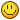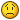# [HELP] attachToBones* get attached element's rotation

## Recommended Posts

Hi everyone!, I have a query regarding this script. I am wanting to rotate an object obtaining its rotation through the function getElementBoneAttachmentDetails and it returns a matrix, of which I do not know how to use it. Would you give me a guide to try to understand?

I don't know how to get the integers rx, ry, rz of the rotation of the object through a matrix

Thanks for the help

Although have no experience with matrix and I'm a noob in mathematicsI have found something interesting in python and convert it to Lua, not sure it will work try converting the matrix with this function:

```function convertRotationMatrixToEulerAngles(matrix)
local roll, pitch, yaw = false, false, false;
local pi = math.pi;
local atan2 = math.atan2;
local m_cos = math.cos;
local m_sin = math.sin;
if matrix then
local m11, m12, m13 = matrix, matrix, matrix;
local m21, m22, m23 = matrix, matrix, matrix;
local m31, m32, m33 = matrix, matrix, matrix;
if m31 ~= 1 and m31 ~= -1 then
pitch_1 = -1*m_sin(m31);
pitch_2 = pi - pitch_1;
roll_1 = m_atan2(m32/m_cos(pitch_1), m33/m_cos(pitch_1));
roll_2 = m_atan2(m33/m_cos(pitch_2), m33/m_cos(pitch_2));
yaw_1 = m_atan2(m21/m_cos(pitch_1), m11/m_cos(pitch_1));
yaw_2 = m_atan2(m21/m_cos(pitch_2), m11/m_cos(pitch_2));
pitch = pitch_1;
roll = roll_1;
yaw = yaw_1;
else
if m31 == -1 then
pitch = pi/2;
roll = yaw + m_atan2(m12, m13);
else
pitch = -pi/2;
roll = -1*yaw + m_atan2(-1*m12,-1*m13);
end
yaw = 0;
end
roll = roll*180/pi;
pitch = pitch*180/pi;
yaw = yaw*180/pi;
return roll, pitch, yaw;
end
end```

It will return (in theory) rotX, rotY, rotZ in degrees

Hope that helps!

•1
• Moderators

It isn't a matrix, 6-7-8th arguments are rx, ry, rz .• Patrick changed the title to [HELP] bone_attach get attached element's rotation
2 hours ago, Patrick said:

It isn't a matrix, 6-7-8th arguments are rx, ry, rz .

He’s talking about attachToBones I have a pm from him also if you visit the link it’s attachToBones

@Tekken Thank you very much for your help! I have used its helpful, but when obtaining the data it gives me wrong numbers. If I rotate X = 90, I should rotate the same with the matrix but it returns other numbersAs I said I have not tested it, may I know what rotx roty and rotz you enter and what you gen when converting the matrix using this function?

I use this code to do what I want

```exports.bone_attach:setElementBoneRotationOffset(object, 180, 40, 0)

local data = exports.bone_attach:getElementBoneAttachmentDetails(object)
local rx, ry, rz = convertRotationMatrixToEulerAngles(data)
print(rx, ry, rz) -- return -140, -5.3751111671239e-15, 180```
• Patrick changed the title to [HELP] attachToBones* get attached element's rotation

## Create an account

Register a new account